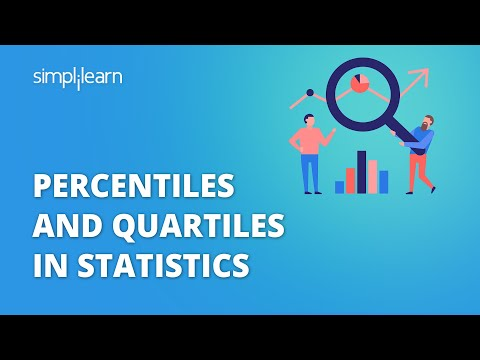### Tutorial PlaylistStatistics is a branch of mathematics that employs quantified models, representations, and summaries to analyze a set of experimental data or real-world studies. Statistics is the study of methods for gathering, reviewing, analyzing, and drawing conclusions from data. In this tutorial, you will explore percentiles in statistics.

In this tutorial, you will look into-

• What is a Percentile in Statistics?
• How to Calculate Percentile?

## What Is a Percentile in Statistics?

In statistics, a percentile is a term that describes how a score compares to other scores from the same set. While there is no universal definition of percentile, it is commonly expressed as the percentage of values in a set of data scores that fall below a given value.

Percentiles show how a given value compares to others. The general rule is that if a value is in the kth percentile, it is greater than K per cent of the total values. Now, take a look at how this information can be useful.

Raw test scores are frequently uninformative. When you get a score on the SAT, CAT, or GRE, the units themselves are meaningless. A total CAT score of 120 isn't necessarily significant. Instead, you're more interested in knowing what percentage of test-takers you outperformed. A total score of 120 on the CAT is roughly in the 90th percentile. Congratulations, you scored better than 90% of the other test-takers. Only 10% scored better than you.

#### Data Analyst Master's Program

In Collaboration With IBM## How to Calculate Percentile?

You can calculate percentiles in statistics using the following formula:For example:

Imagine you have the marks of 20 students. Now, try to calculate the 90th percentile.Step 1: Arrange the score in ascending order.Step 2: Plug the values in the formula to find n.P90 = 94 means that 90% of students got less than 94 and 10% of students got more than 94

Let’s look at another way how you can find the percentile in statistics.

Suppose you want to find the percentile mark of 78 marks in the data set.

#### Data Analytics Free Course

Start Learning Today's Most In-Demand SkillsStep 1: Sort the marks in ascending order.Step 2: Substitute the value in the formula.P = 60 means that 78 marks point to the 60th percentile in the dataset.

Looking forward to a career in Data Analytics? Check out the Data Analytics Bootcamp and get certified today.

## Conclusion

With this, you come to an end of this ‘percentile in statistics’ tutorial. You have learnt about what is percentile and how you can calculate the percentile of any data set with the help of a formula.

Statistics is often the most fundamental of skills that are required for a career in Analytics. If you are keen on mastering key statistical skills and other vital analytical skills needed for such a career, you ought to explore Simplilearn’s Post Graduate Program in Data Analytics. This bootcamp-style course is a 8-month intensive program delivered in collaboration with Purdue University and IBM. It features applied training in today’s top Data Analytics topics and skills, Masterclasses from Purdue faculty and IBM, and multiple projects, case studies and a capstone project to help you prove your practical expertise of the skills learnt to future employers.

If you have any questions regarding this ‘Percentiles in statistics’ tutorial, do share them in the comment section. Our subject matter expert will respond to your queries. Happy learning!Simplilearn